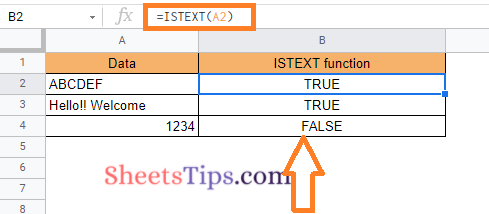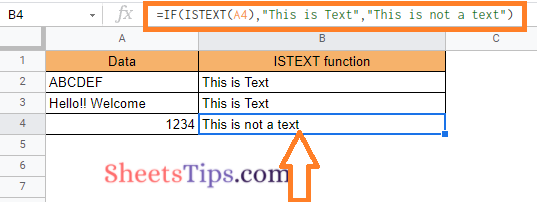# How to Use the ISTEXT Function in Google Sheets? (With Example)

The ISTEXT function in google sheets checks cells for text value and returns TRUE or FALSE. This function can be useful when you have to validate the data which contains only text.

## What Is ISTEXT Function in Google Sheets?

ISTEXT is a very straightforward function. If the given cell is text, you will get a “TRUE” response, and if it’s not a text, you will get a “FALSE” response.

It is one of google sheets functions that examine the data in a cell to identify what type of data it is — and whether it’s the correct form of data.

ISTEXT function will return only TRUE or FALSE as the response. This can be used in the IF function for more validation part.

Syntax:

=ISTEXT(data)

In the above syntax,

• ISTEXT – This is the keyword for the ISTEXT function in google sheets
• data – Is the cell that you have to validate if the given data is text or not.

## How To Use ISTEXT Function in Google Sheets?

Now, let’s see an example of how to use the ISTEXT function in google sheets.In the above example,

We are checking if the cell is having text or not with the formula =ISTEXT(A2).

We are having data with “ABCDEF” and “Hello!! Welcome” which is considered as the text, and the response is TRUE. The cell having “1234” which is numeric, is not considered as text, and the response is FALSE.

## Using ISTEXT with the IF Function in Google Sheets?

We could validate the ISTEXT with the IF function in google sheets.

Formula:

=IF(ISTEXT(data),”condition_1″,”condition_2″)

In the above formula,

• IF – If is the keyword for calling the IF statement function in google sheets
• ISTEXT – This is the keyword for the ISTEXT function in google sheets
• data – This is the cell that you have to validate if the given data is text or not
• condition_1 – If the validation is TRUE(cell is having text) then executes given condition here
• condition_2 – If the validation is FALSE(cell is not having text) then executes given condition here

Let’s see an example of how to use the ISTEXT function inside the IF condition.In the above example,

We are checking if the cell is having text or not using if function with the formula –

=IF(ISTEXT(A4), “This is Text”, “This is not a text”)

We are having data with “ABCDEF” and “Hello!! Welcome” which is considered as the text, and the response is “This is Text”. The cell having “1234” which is numeric, is not considered as text, and the response is “This is not a text”.

Using the IF statement with the ISTEXT function makes it clear to the user that they have entered incorrect content. This can be useful when gathering any kind of information.

## ISTEXT Similar Functions in Google Sheets

Similar to ISTEXT Function, we also have functions like,

• ISNUMBER – Returns TRUE, if the data is having a numeric value.
• ISDATE – Returns TRUE, if the data is having the date
• ISURL – Returns TRUE, if the data is having a URL
• ISEVEN – Returns TRUE, if the data is having an even number value
• ISODD – Returns TRUE, if the data is having an odd number value

All of these are text validation sequences designed to help you determine whether the correct content has been entered into a spreadsheet.